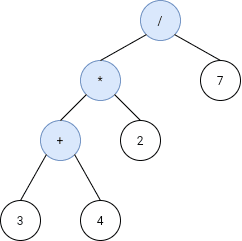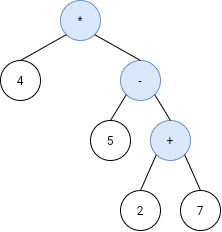# Question

Formatted question description: https://leetcode.ca/all/1628.html

Given the postfix tokens of an arithmetic expression, build and return the binary expression tree that represents this expression.

Postfix notation is a notation for writing arithmetic expressions in which the operands (numbers) appear before their operators. For example, the postfix tokens of the expression 4*(5-(7+2)) are represented in the array postfix = ["4","5","7","2","+","-","*"].

The class Node is an interface you should use to implement the binary expression tree. The returned tree will be tested using the evaluate function, which is supposed to evaluate the tree's value. You should not remove the Node class; however, you can modify it as you wish, and you can define other classes to implement it if needed.

A binary expression tree is a kind of binary tree used to represent arithmetic expressions. Each node of a binary expression tree has either zero or two children. Leaf nodes (nodes with 0 children) correspond to operands (numbers), and internal nodes (nodes with two children) correspond to the operators '+' (addition), '-' (subtraction), '*' (multiplication), and '/' (division).

It's guaranteed that no subtree will yield a value that exceeds 109 in absolute value, and all the operations are valid (i.e., no division by zero).

Follow up: Could you design the expression tree such that it is more modular? For example, is your design able to support additional operators without making changes to your existing evaluate implementation?

Example 1:Input: s = ["3","4","+","2","*","7","/"]
Output: 2
Explanation: this expression evaluates to the above binary tree with expression ((3+4)*2)/7) = 14/7 = 2.


Example 2:Input: s = ["4","5","2","7","+","-","*"]
Output: -16
Explanation: this expression evaluates to the above binary tree with expression 4*(5-(2+7)) = 4*(-4) = -16.


Constraints:

• 1 <= s.length < 100
• s.length is odd.
• s consists of numbers and the characters '+', '-', '*', and '/'.
• If s[i] is a number, its integer representation is no more than 105.
• It is guaranteed that s is a valid expression.
• The absolute value of the result and intermediate values will not exceed 109.
• It is guaranteed that no expression will include division by zero.

# Algorithm

Diff from 1597-Build-Binary-Expression-Tree-From-Infix-Expression is, there is no brackets () in expression, so making it easier to evaluate.

The analysis of postfix expressions is relatively easy. As for the evaluate method, it can be implemented recursively.

If the current node is a value, the value is returned directly, otherwise the left and right subtrees are calculated recursively, and then the operation of the tree root is calculated, and finally returned.

### Follow up: Could you design the expression tree such that it is more modular? For example, is your design able to support additional operators without making changes to your existing evaluate implementation?

Just separate Node and Tree into 2 classes, then operators are on each Node, while Tree will not be affect.

# Code

• 

public class Design_an_Expression_Tree_With_Evaluate_Function {

/**
* This is the TreeBuilder class.
* You can treat it as the driver code that takes the postinfix input
* and returns the expression tree represnting it as a Node.
*/

class TreeBuilder {
Node buildTree(String[] postfix) {
Stack<Node> stack = new Stack<>();
for (String s : postfix) {
switch (s) {
case "+":
case "-":
case "*":
case "/":
stack.push(new Node(s, stack.pop(), stack.pop()));
break;
default:
stack.push(new Node(s, null, null));
}
}
return stack.peek();
}
};

/**
* This is the interface for the expression tree Node.
* You should not remove it, and you can define some classes to implement it.
*/

class Node {
String val;
Node left, right;
Node(String val, Node left, Node right) {
this.val = val;
this.left = left;
this.right = right;
}

public int evaluate() {
if (left == null && right == null)
return Integer.valueOf(val);

int l = left.evaluate();
int r = right.evaluate();

switch (val) {
case "+":
return l+r;
case "-":
return r-l;
case "*":
return l*r;
case "/":
return r/l;
}
return -1;
}
};

/**
* Your TreeBuilder object will be instantiated and called as such:
* TreeBuilder obj = new TreeBuilder();
* Node expTree = obj.buildTree(postfix);
* int ans = expTree.evaluate();
*/

}

############

/**
* This is the interface for the expression tree Node.
* You should not remove it, and you can define some classes to implement it.
*/

abstract class Node {
public abstract int evaluate();
protected String val;
protected Node left;
protected Node right;
};

class MyNode extends Node {
public MyNode(String val) {
this.val = val;
}

public int evaluate() {
if (isNumeric()) {
return Integer.parseInt(val);
}
int leftVal = left.evaluate();
int rightVal = right.evaluate();
if (Objects.equals(val, "+")) {
return leftVal + rightVal;
}
if (Objects.equals(val, "-")) {
return leftVal - rightVal;
}
if (Objects.equals(val, "*")) {
return leftVal * rightVal;
}
if (Objects.equals(val, "/")) {
return leftVal / rightVal;
}
return 0;
}

public boolean isNumeric() {
for (char c : val.toCharArray()) {
if (!Character.isDigit(c)) {
return false;
}
}
return true;
}
}

/**
* This is the TreeBuilder class.
* You can treat it as the driver code that takes the postinfix input
* and returns the expression tree represnting it as a Node.
*/

class TreeBuilder {
Node buildTree(String[] postfix) {
Deque<MyNode> stk = new ArrayDeque<>();
for (String s : postfix) {
MyNode node = new MyNode(s);
if (!node.isNumeric()) {
node.right = stk.pop();
node.left = stk.pop();
}
stk.push(node);
}
return stk.peek();
}
};

/**
* Your TreeBuilder object will be instantiated and called as such:
* TreeBuilder obj = new TreeBuilder();
* Node expTree = obj.buildTree(postfix);
* int ans = expTree.evaluate();
*/

• /**
* This is the interface for the expression tree Node.
* You should not remove it, and you can define some classes to implement it.
*/

class Node {
public:
virtual ~Node() {};
virtual int evaluate() const = 0;

protected:
string val;
Node* left;
Node* right;
};

class MyNode : public Node {
public:
MyNode(string val) {
this->val = val;
}

MyNode(string val, Node* left, Node* right) {
this->val = val;
this->left = left;
this->right = right;
}

int evaluate() const {
if (!(val == "+" || val == "-" || val == "*" || val == "/")) return stoi(val);
auto leftVal = left->evaluate(), rightVal = right->evaluate();
if (val == "+") return leftVal + rightVal;
if (val == "-") return leftVal - rightVal;
if (val == "*") return leftVal * rightVal;
if (val == "/") return leftVal / rightVal;
return 0;
}
};

/**
* This is the TreeBuilder class.
* You can treat it as the driver code that takes the postinfix input
* and returns the expression tree represnting it as a Node.
*/

class TreeBuilder {
public:
Node* buildTree(vector<string>& postfix) {
stack<MyNode*> stk;
for (auto s : postfix) {
MyNode* node;
if (s == "+" || s == "-" || s == "*" || s == "/") {
auto right = stk.top();
stk.pop();
auto left = stk.top();
stk.pop();
node = new MyNode(s, left, right);
} else {
node = new MyNode(s);
}
stk.push(node);
}
return stk.top();
}
};

/**
* Your TreeBuilder object will be instantiated and called as such:
* TreeBuilder* obj = new TreeBuilder();
* Node* expTree = obj->buildTree(postfix);
* int ans = expTree->evaluate();
*/

• '''
>>> "123".isdigit()
True
>>> "123abc".isdigit()
False
'''

"""
This is the interface for the expression tree Node.
You should not remove it, and you can define some classes to implement it.
"""

import abc
from abc import ABC, abstractmethod

class Node(ABC):
@abstractmethod
def evaluate(self) -> int:
pass

class MyNode(Node):
def __init__(self, val):
self.val = val
self.left = None
self.right = None

def evaluate(self) -> int:
x = self.val
if x.isdigit():
return int(x)

left, right = self.left.evaluate(), self.right.evaluate()
if x == '+':
return left + right
if x == '-':
return left - right
if x == '*':
return left * right
if x == '/':
return left // right

"""
This is the TreeBuilder class.
You can treat it as the driver code that takes the postinfix input
and returns the expression tree represnting it as a Node.
"""

class TreeBuilder(object):
def buildTree(self, postfix: List[str]) -> 'Node':
stk = []
for s in postfix:
node = MyNode(s)
if not s.isdigit():
node.right = stk.pop()
node.left = stk.pop()
stk.append(node)
return stk[-1]

"""
Your TreeBuilder object will be instantiated and called as such:
obj = TreeBuilder();
expTree = obj.buildTree(postfix);
ans = expTree.evaluate();
"""